RRB Group D Mock Test - 23

# RRB Group D Mock Test - 23

Test Description

## 100 Questions MCQ Test RRB (Group D) - Mock Tests & Previous Year Papers | RRB Group D Mock Test - 23

RRB Group D Mock Test - 23 for Railways 2022 is part of RRB (Group D) - Mock Tests & Previous Year Papers preparation. The RRB Group D Mock Test - 23 questions and answers have been prepared according to the Railways exam syllabus.The RRB Group D Mock Test - 23 MCQs are made for Railways 2022 Exam. Find important definitions, questions, notes, meanings, examples, exercises, MCQs and online tests for RRB Group D Mock Test - 23 below.
Solutions of RRB Group D Mock Test - 23 questions in English are available as part of our RRB (Group D) - Mock Tests & Previous Year Papers for Railways & RRB Group D Mock Test - 23 solutions in Hindi for RRB (Group D) - Mock Tests & Previous Year Papers course. Download more important topics, notes, lectures and mock test series for Railways Exam by signing up for free. Attempt RRB Group D Mock Test - 23 | 100 questions in 90 minutes | Mock test for Railways preparation | Free important questions MCQ to study RRB (Group D) - Mock Tests & Previous Year Papers for Railways Exam | Download free PDF with solutions
 1 Crore+ students have signed up on EduRev. Have you?
RRB Group D Mock Test - 23 - Question 1

### The following question consists of two words each that have a certain relationship to each other, followed by pairs of words. Select the lettered pair that has the same relationship as the original pair of words. Fritter : Squander :: ?

Detailed Solution for RRB Group D Mock Test - 23 - Question 1

Squander is synonym of Fritter.

Similarly, Invigorate is synonym of Rejuvenate.

RRB Group D Mock Test - 23 - Question 2

### The following question consists of a pair of letter - groups that have a certain relationship to each other, followed by four other pairs of letter – groups given as alternative. Select the pair in which the letter – groups are similarly related as in the given pair. ABCD : MPSV : : EFGH : ?

Detailed Solution for RRB Group D Mock Test - 23 - Question 2

The pattern is as follows –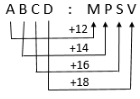Similarly,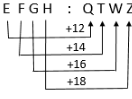RRB Group D Mock Test - 23 - Question 3

### The following question consists of a pair of numbers that have a certain relationship to each other, followed by four other numbers given as alternative. Select the option in which the numbers are similarly related as in the given pair. 13 : 172 :: 23 : ?

Detailed Solution for RRB Group D Mock Test - 23 - Question 3

The given relation is:

13 : (13)2 + 3 = 13 : 172

Similarly,

23 : (23)2 + 3 = 23 : 532

RRB Group D Mock Test - 23 - Question 4

Which one set of letters when sequentially placed in the gaps in the given letter series shall complete it?

_aba_aab_aaa_aa

Detailed Solution for RRB Group D Mock Test - 23 - Question 4

The given cyclic order series follows the below pattern:

‘aabaa’

aabaa|aabaa|aabaa

RRB Group D Mock Test - 23 - Question 5

The following question consists various terms of letter series with two terms missing as shown by (?). Choose the missing terms out of the given alternatives.

A, B, B, D, C, F, D, H, E, ?, ?

Detailed Solution for RRB Group D Mock Test - 23 - Question 5

The given sequence is a combination of two series –

(I) 1st, 3rd, 5th, 7th, 9th, 11th terms, i.e., A, B, C, D, E

(II) 2nd, 4th, 6th, 8th, 10th terms, i.e., B, D, F, H

Therefore in series (I) next term will be F and in series (II) next term will be J.

Therefore, option (a) is the right answer.

RRB Group D Mock Test - 23 - Question 6

In a certain code language,

‘trust is good’ is written as ‘lo ma sa’,

‘people trust daily’ is written as ‘fa sa re’,

‘make good friends’ is written as ‘te ma co’.
Q.How is ‘people’ written in that code language?

Detailed Solution for RRB Group D Mock Test - 23 - Question 6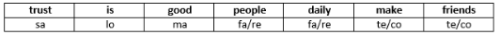RRB Group D Mock Test - 23 - Question 7

In a certain code language,

‘trust is good’ is written as ‘lo ma sa’,

‘people trust daily’ is written as ‘fa sa re’,

‘make good friends’ is written as ‘te ma co’.

Q.The code ‘te’ stands for which word?

Detailed Solution for RRB Group D Mock Test - 23 - Question 7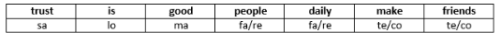RRB Group D Mock Test - 23 - Question 8

If ‘+’ means ‘÷’, ‘÷’ means ‘×’, ‘×’ means ‘-‘, ‘-‘ means ‘+’, then what is the value of:

34 + 17 ÷ 9 × 3 – 54 = ?

Detailed Solution for RRB Group D Mock Test - 23 - Question 8

As, ‘+’ means ‘÷’, ‘÷’ means ‘×’, ‘×’ means ‘-‘, ‘-‘ means ‘+’

Therefore, the given expression: 34 + 17 ÷ 9 × 3 – 54 can be rewritten as:

34 ÷ 17 × 9 – 3 + 54 = 69.

RRB Group D Mock Test - 23 - Question 9

Surekha’s father’s mother’s son’s wife is related to Surekha as?

Detailed Solution for RRB Group D Mock Test - 23 - Question 9

As per the given equation, there can be two cases as shown below. Hence, Surekha’s father’s mother’s son’s wife may be Surekha’s mother or aunt. Therefore, relationship cannot be determined.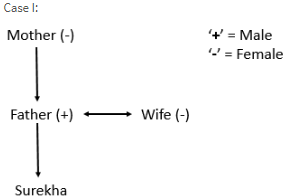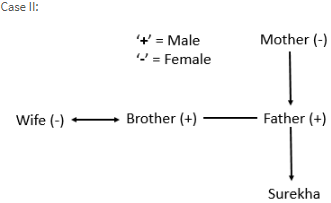RRB Group D Mock Test - 23 - Question 10

Pointing to a lady in a photograph, Manisha said “She is the mother of Srishti, whose father is my son”. How is the lady in the picture related to Manisha?

Detailed Solution for RRB Group D Mock Test - 23 - Question 10

The lady in the picture is daughter – in – law of Manisha.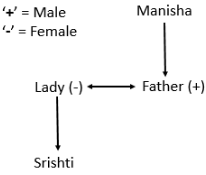RRB Group D Mock Test - 23 - Question 11

A man starts walking straight towards east. After walking 55 metres, he turns left and walks 20 metres straight. Again he turns left, walks a distance of 35 metres straight, again turns left and walks a distance of 20 metres. How far is he from the starting point?

Detailed Solution for RRB Group D Mock Test - 23 - Question 11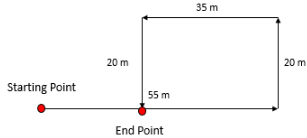RRB Group D Mock Test - 23 - Question 12

The door of Shubham's house faces East. From the back side of his house, he walks straight 50 m, then turns to the right and walks 50 m again. Finally, he turns towards left and stops after walking 25 m. Now, Shubham is in which direction from the starting point?

Detailed Solution for RRB Group D Mock Test - 23 - Question 12

As door of Shubham’s house faces East and he starts walking from the back side of his house, that means he starts walking in West direction.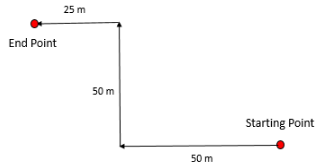Therefore, he is in north – west direction from the starting point.

RRB Group D Mock Test - 23 - Question 13

Starting from a point A, Vinod walked 20 metres towards South. He turned left and walked 30 metres. He then turned left and walked 20 metres. He again turned left and walked 40 metres and reached a point S. How far and in which direction is the point S from the point A?

Detailed Solution for RRB Group D Mock Test - 23 - Question 13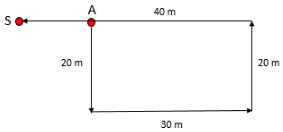RRB Group D Mock Test - 23 - Question 14

In the given figure, which number represents students who are activists, but not youth icons?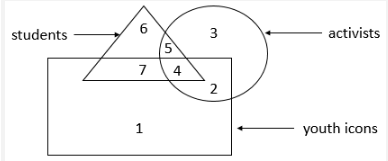Detailed Solution for RRB Group D Mock Test - 23 - Question 14

The required region is the one which is common to triangle and circle but not rectangle i.e., 5.

RRB Group D Mock Test - 23 - Question 15

Which one of the following figures represents the given statements?

All persons know English. Few of them know Sanskrit also. Those who know Sanskrit also know German.

Detailed Solution for RRB Group D Mock Test - 23 - Question 15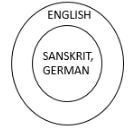RRB Group D Mock Test - 23 - Question 16

In each of the following questions, some statements are given followed by three conclusions numbered I, II and III. You have to take the given statements to be true even if they seem to be at variance with commonly known facts and then decide which of the given conclusions logically follows from the given statements disregarding commonly known facts.

Statements:

Some ships are boats.

All boats are raft.

Conclusions:

I. Some raft are ships.

II. All ships are raft.

III. All boats are ships.

Detailed Solution for RRB Group D Mock Test - 23 - Question 16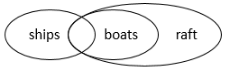Conclusion I: Some raft are ships: FOLLOWS: It is definite.

Conclusion II: All ships are raft: Not Follows: It is not definite.

Conclusion III: All boats are ships: Not Follows: It is not definite.

RRB Group D Mock Test - 23 - Question 17

Statements:

All boxes are hammers.

No rings are hammers.

All nails are rings.

Conclusions:

I. No nails are boxes.

II. No hammers are nails.

III. No boxes are rings.

Detailed Solution for RRB Group D Mock Test - 23 - Question 17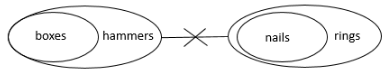Conclusion I: No nails are boxes: FOLLOWS: It is definite.

Conclusion II: No hammers are nails: FOLLOWS: It is definite.

Conclusion III: No boxes are rings: FOLLOWS: It is definite.

RRB Group D Mock Test - 23 - Question 18

Each question given below consists of a statement, followed by two arguments I and II. You have to decide which of the arguments is a strong argument and which is a weak argument.

Statement:

Should India encourage exports, when most things are insufficient for internal use itself?

Arguments:

(I) Yes. We have to earn foreign exchange to pay for our imports.

(II) No. Even selective encouragement would lead to shortages.

Detailed Solution for RRB Group D Mock Test - 23 - Question 18

Clearly, India can export only the surplus and that which can be saved after fulfilling its own needs, to pay for its imports. Encouragement to export cannot lead to shortages as it shall provide the resources for imports. So, only argument I holds.

RRB Group D Mock Test - 23 - Question 19

Each question given below consists of a statement, followed by two arguments I and II. You have to decide which of the arguments is a strong argument and which is a weak argument.

Statement:

Should India make efforts to harness solar energy to fulfil its energy requirements?

Arguments:

(I) Yes, Most of the energy sources used at present is exhaustible.

(II) No. Harnessing solar energy requires a lot of capital, which India lacks in.

Detailed Solution for RRB Group D Mock Test - 23 - Question 19

Clearly, harnessing solar energy will be helpful as it is an inexhaustible resource unlike other resources. So, argument I holds. But argument II is vague as solar energy is the cheapest form of energy.

RRB Group D Mock Test - 23 - Question 20

Find the odd one out from the following options.

(64, 83) (100, 121) (169, 196) (36, 49)

Detailed Solution for RRB Group D Mock Test - 23 - Question 20

In all others except (64, 83) the two given numbers are squares of consecutive natural numbers.

RRB Group D Mock Test - 23 - Question 21

Find the odd one out from the following options.

Detailed Solution for RRB Group D Mock Test - 23 - Question 21

Except Param Veer Chakra, all others are civilian awards.

RRB Group D Mock Test - 23 - Question 22

In a row of students, Ricky is 10th to the left of Raj, who is 30th from the left end. If Raj is exactly in the middle of Ricky and Sanket, then find -
Q. How many minimum number of students is possible in that row?

Detailed Solution for RRB Group D Mock Test - 23 - Question 22

From the given information we get position of Sanket as 40th. Therefore, minimum number of students possible in the row = 40.

RRB Group D Mock Test - 23 - Question 23

In a row of students, Ricky is 10th to the left of Raj, who is 30th from the left end. If Raj is exactly in the middle of Ricky and Sanket, then find -
Q. What is the total strength?

Detailed Solution for RRB Group D Mock Test - 23 - Question 23

No such information is given in the question from which we can calculate the total strength.

RRB Group D Mock Test - 23 - Question 24

In each of the following question, four words are given. Which of them will come at the third place if all of them are arranged alphabetically as in a dictionary.

Detailed Solution for RRB Group D Mock Test - 23 - Question 24

The arrangement of given words as per dictionary is – Delight, Demon, Disarm, Dislocate.

Thus, third word in alphabetical order as in dictionary will be: Disarm

RRB Group D Mock Test - 23 - Question 25

In each of the following question, four words are given. Which of them will come at the second place if all of them are arranged alphabetically as in a dictionary.

Detailed Solution for RRB Group D Mock Test - 23 - Question 25

The arrangement of given words as per dictionary is – Fantastic, Fantasy, Farming, Fathom.

Thus, second word in alphabetical order as in dictionary will be: Fantasy

RRB Group D Mock Test - 23 - Question 26

A, B and C complete a piece of work in 30, 15 and 10 days respectively, while working individually. How much time will they take to complete the same work while working together?

Detailed Solution for RRB Group D Mock Test - 23 - Question 26

As per the formula,

Required days = (30 * 15 * 10) / (30*15 + 15*10 + 10*30) = 4500 / 900 = 5 days

RRB Group D Mock Test - 23 - Question 27

If 6 men and 8 boys can do a piece of work in 10 days while 26 men and 48 boys can do the same piece of work in 2 days, the time taken by 15 men and 20 boys in doing the same work will be

Detailed Solution for RRB Group D Mock Test - 23 - Question 27

Let work done by 1 man in 1 day be x

Also, work done by 1 boy in 1 day be y

Then, 6x + 8y = 1/10

Also, 26x + 48y = 1/2

Solving these two equations, we get,

x = 1/100 and y = 1/200

∴ 1-day work of 15 men and 20 boys = 15/100 + 20/200 = 1/4

=> 15 men and 20 boys can do the work in 4 days.

RRB Group D Mock Test - 23 - Question 28

If x/y = 4/5, find the value of (3x + 4y) / (4x + 3y)

Detailed Solution for RRB Group D Mock Test - 23 - Question 28

(3x + 4y) / (4x + 3y) = (3x/y + 4) / (4x/y + 3) [Dividing numerator and denominator by y]

= (3*(4/5) + 4) / (4*(4/5) + 3) = 32/31

RRB Group D Mock Test - 23 - Question 29

A triangle is having sides as 50m, 78m and 112m. Find the length of perpendicular on the side 112m.

Detailed Solution for RRB Group D Mock Test - 23 - Question 29

Here, a = 50m, b = 78m and c = 112m

S = (a + b + c)/2 = (50 + 78 + 112)/2 = 120m

∴ S – a = 120 – 50 = 70m

S – b = 120 – 78 = 42m

S – c = 120 – 112 = 8m

Now, Area = √[s*(s-a)(s-b)(s-c)] = √(120 * 70 * 42 * 8) = 1680m2

Also, Area = (base * perpendicular) / 2

1680 = (112 * P) / 2

=> P = 30m

RRB Group D Mock Test - 23 - Question 30

A circle and a rectangle have same perimeter. The side of rectangle are 18cm * 26cm. Find the area of circle.

Detailed Solution for RRB Group D Mock Test - 23 - Question 30

Perimeter of circle = Perimeter of rectangle

i.e., 2πr = 2*(l + b)

2πr = 2*(18 + 26)

2 * 22/7 * r = 88

=> r = 14

∴ Area of circle = πr2 = π*142 = 616cm2

RRB Group D Mock Test - 23 - Question 31

If the area of a circle decreases by 36%, then the radius of the circle decreases by

Detailed Solution for RRB Group D Mock Test - 23 - Question 31

A = πr2

The area is decreased by 36%

=> remaining area = 0.64 A

Suppose the remaining radius = x times of r

∴ 0.64A = π(xr)2 = x2πr2

0.64 A = x2 * A

=> x2 = 0.64

i.e., x = 0.8 times of r is remaining

RRB Group D Mock Test - 23 - Question 32

Find the compound interest on Rs.25625 for 1 year at 16% per annum, compounded half yearly.

Detailed Solution for RRB Group D Mock Test - 23 - Question 32

Principal (P) = Rs.25625

Rate (r) = 16/2 = 8%

Time = 1 year = 2 half years

∴ A = 25625 * (1 + 8/100)2 = 25625 * (27/25) 2

A = Rs.29889

=> CI = A – P = 29889 – 25625 = Rs.4264

RRB Group D Mock Test - 23 - Question 33

There are 30 students in a class. Average age of first 10 students is 12.5 years and average age of other 20 students is 13.1 years. Find the average age of whole class.

Detailed Solution for RRB Group D Mock Test - 23 - Question 33

Sum of ages of first 10 students = 10 * 12.5 = 125

And, Sum of ages of other 20 students = 20 * 13.1 = 262

=> Average age of whole class = (125 + 262) / 30 = 387/30 = 12.9 years

RRB Group D Mock Test - 23 - Question 34

The average of 10 numbers is 40.2. Later it is found that two numbers have been wrongly copied. The first added is 18 greater than the actual number and the second number added is 13 instead of 31. Find the correct average.

Detailed Solution for RRB Group D Mock Test - 23 - Question 34

Sum of 10 numbers = 10 * 40.2 = 402

1st number added is 18 more than actual number, So, 18 must be subtracted

Also, 2nd number added is 13 instead of 31, So, 13 should be subtracted and 31 should be added

=> Actual sum of 10 numbers = 402 – 18 – 13 + 31 = 402

=> Actual average = 402 / 10 = 40.2

RRB Group D Mock Test - 23 - Question 35

If the CP of 15 Pen is equal to SP of 20 pen, Find the loss percent.

Detailed Solution for RRB Group D Mock Test - 23 - Question 35

Let CP of 1 Pen be x

Since SP of 20 Pen = CP of 15 Pen

i.e., SP of 1 Pen = CP of (15/20) Pen

∴ SP of 1 Pen = (15/20)x = (3/4)x

=> Loss = CP – SP = x - (3/4)x = x/4

=> Loss % = ((x/4)/x) * 100 = 25%

RRB Group D Mock Test - 23 - Question 36

A single discount equal to two consecutive discounts of 20% and 10% is

Detailed Solution for RRB Group D Mock Test - 23 - Question 36

Let the Marked Price be Rs.x

After a consecutive discount of 20% and 10%,

Selling Price = x * (80/100) * (90/100) = (72/100) * x

=> Single discount = x - (72/100) * x = (28/100) * x = 28%

RRB Group D Mock Test - 23 - Question 37

Find the unit digit of 263.

Detailed Solution for RRB Group D Mock Test - 23 - Question 37

Here unit digits will repeat as 2, 4, 8, 6 after every four intervals till 60. Next 61 will be 2, 62 will be 4 and 63 will be 8.

So, the unit digit of 263 = 8

RRB Group D Mock Test - 23 - Question 38

3/7 of 4/5 of 5/3 of 7/5 of 900 is

Detailed Solution for RRB Group D Mock Test - 23 - Question 38

(3/7) * (4/5) * (5/3) * (7/5) * 900 = (4/5) * 900 = 720

RRB Group D Mock Test - 23 - Question 39

Find the missing term in the following number series.
Q. 12, 12, 24, 72, 288, ?

Detailed Solution for RRB Group D Mock Test - 23 - Question 39

12(* 1 =), 12(* 2 =), 24(* 3 =), 72(* 4 =), 288(* 5 =), 1440

RRB Group D Mock Test - 23 - Question 40

Find the missing term in the following number series.
Q.13, 22, 32, 43, 55, ?

Detailed Solution for RRB Group D Mock Test - 23 - Question 40

13(+ 9 =), 22(+ 10 =), 32(+ 11 =), 43(+ 12 =), 55(+ 13 =), 68

RRB Group D Mock Test - 23 - Question 41

Find the missing term in the following number series.
Q.15, 30, 10, 40, 8, ?

Detailed Solution for RRB Group D Mock Test - 23 - Question 41

15(* 2 =), 30(÷ 3 =), 10(* 4 =), 40(÷ 5 =), 8(* 6 =), 48

RRB Group D Mock Test - 23 - Question 42

In the table given below there are four mixtures of Wine and water. Percentage of Wine and Total Quantity of mixture is given for each mixture.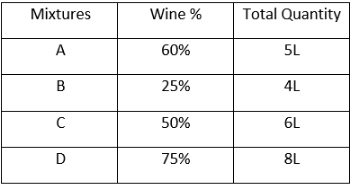Q. If A and B are mixed, Find the total quantity of water in the new mixture

Detailed Solution for RRB Group D Mock Test - 23 - Question 42

Total quantity of water (A + B) = 40% of 5L + 75% of 4L = 2L + 3L = 5L

RRB Group D Mock Test - 23 - Question 43

In the table given below there are four mixtures of Wine and water. Percentage of Wine and Total Quantity of mixture is given for each mixture.Q. Sum of Quantity of water in C and Quantity of Wine in D is

Detailed Solution for RRB Group D Mock Test - 23 - Question 43

50% of 6L + 75% of 8L = 3L + 6L = 9L

RRB Group D Mock Test - 23 - Question 44

In the table given below there are four mixtures of Wine and water. Percentage of Wine and Total Quantity of mixture is given for each mixture.Q. Total Quantity of Wine in all four mixtures is

Detailed Solution for RRB Group D Mock Test - 23 - Question 44

60% of 5L + 25% of 4L + 50% of 6L + 75% of 8L = 3 + 1 + 3 + 6 = 13L

RRB Group D Mock Test - 23 - Question 45

If + means –, * means +, ÷ means * and – means ÷, then solve the following
Q. 64 – 8 * 2 ÷ 6 = ?

Detailed Solution for RRB Group D Mock Test - 23 - Question 45

64 – 8 * 2 ÷ 6 = 64 ÷ 8 + 2 * 6 = 8 + 12 = 20

RRB Group D Mock Test - 23 - Question 46

If + means –, * means +, ÷ means * and – means ÷, then solve the following
Q. 3 * 36 – 6 ÷ 2 + 3 = ?

Detailed Solution for RRB Group D Mock Test - 23 - Question 46

3 * 36 – 6 ÷ 2 + 3 = 3 + 36 ÷ 6 * 2 – 3 = 3 + 6 * 2 – 3 = 3 + 12 – 3 = 12

RRB Group D Mock Test - 23 - Question 47

A man rows a boat in a river, 12 km upstream in 6 hours and 28 km downstream in 4 hours. Find the speed of the boat in still water.

Detailed Solution for RRB Group D Mock Test - 23 - Question 47

Downstream speed = 28/4 = 7km/hr

Upstream speed = 12/6 = 2km/hr

Suppose, Speed of Boat in still water = x km/hr

And, Speed of Stream of river = y km/hr

Then, x + y = 7

And, x – y = 2

Solving these two equations, we get,

x = 4.5 and y = 2.5

=> Speed of Boat in still water= x = 4.5km/hr

RRB Group D Mock Test - 23 - Question 48

How long does a train 100 m long running at a speed of 60 km/hr takes to cross another train 150 m long running at a speed of 40 km/hr in the same direction?

Detailed Solution for RRB Group D Mock Test - 23 - Question 48

Another train is moving in same direction.

Then, Length to be covered = 100 + 150 = 250m

And Relative Speed = 60 – 40 = 20km/hr

=> Required time = 250 / (20 * 5/18) = (250 * 18) / (20 * 5) = 45 secs

RRB Group D Mock Test - 23 - Question 49

Solve the equation and Find the values of x.

27x3 – 54x2 + 36x – 8 = 0

Detailed Solution for RRB Group D Mock Test - 23 - Question 49

27x3 – 54x2 + 36x – 8 = 0

(3x)3 + 3*(3x)2*(-2) + 3*(3x)*(-2)2 + (-2)3 = 0

(3x – 2)3 = 0 [Using, (a + b)3 = a3 + 3a2b + 3ab2 + b3]

=> 3x – 2 = 0

Hence, x = 2/3

RRB Group D Mock Test - 23 - Question 50

If Sin Ѳ = 12/13, then find the value of Sec Ѳ + Tan Ѳ

Detailed Solution for RRB Group D Mock Test - 23 - Question 50

Since, Sin Ѳ = 12/13

Cos2 Ѳ = 1 – Sin2 Ѳ = 1 – (12/13)2

= 1 – (144/169) = 25/169

=> Cos Ѳ = 5/13

Hence, Sec Ѳ = 1/Cos Ѳ = 1/ (5/13) = 13/5

And, Tan Ѳ = Sin Ѳ/Cos Ѳ = (12/13) / ( 5/13) = 12/5

=> Sec Ѳ + Tan Ѳ = 13/5 + 12/5 = 25/5 = 5

RRB Group D Mock Test - 23 - Question 51

Amino acids are found in

Detailed Solution for RRB Group D Mock Test - 23 - Question 51

Amino acids that are the building blocks of protein that our body can't produce by itself .

RRB Group D Mock Test - 23 - Question 52

Which of the following ore does not contain iron?

RRB Group D Mock Test - 23 - Question 53

Which of the following is the example of emulsion?

RRB Group D Mock Test - 23 - Question 54

Average quantity of blood in Human body?

RRB Group D Mock Test - 23 - Question 55

Unit of Planck’s constant is

Detailed Solution for RRB Group D Mock Test - 23 - Question 55

In SI units, the Planck’s is expressed in joule-seconds (js).

RRB Group D Mock Test - 23 - Question 56

The watermelon model was given by-

Detailed Solution for RRB Group D Mock Test - 23 - Question 56

Thomson's Model of an Atom J. J. Thomson proposed that an atom was a solid sphere of positively charged material and the negatively charged particles, electrons, were embedded in it like the seeds in a watermelon.

RRB Group D Mock Test - 23 - Question 57

The Lassa fever can spread to human through an infected-

Detailed Solution for RRB Group D Mock Test - 23 - Question 57

Lassa fever is an acute and often fatal viral disease, with fever, occurring chiefly in West Africa. It is usually acquired from infected rats.

RRB Group D Mock Test - 23 - Question 58

A boat will submerge when it displaces water equal to its own-

RRB Group D Mock Test - 23 - Question 59

JJ Thompson is credited for the discovery of –

Detailed Solution for RRB Group D Mock Test - 23 - Question 59

In 1897, Thomson set out to prove that the cathode rays produced from the cathode were actually a stream of negatively charged particles called electrons.

RRB Group D Mock Test - 23 - Question 60

Which of the following explains the dual nature of electrons?

Detailed Solution for RRB Group D Mock Test - 23 - Question 60

In 1924, the French physicist, Louis de Broglie suggested that if light has electron, behaves both as a material particle and as a wave.

RRB Group D Mock Test - 23 - Question 61

Gamma rays were discovered by-

Detailed Solution for RRB Group D Mock Test - 23 - Question 61

Paul Villard, a French physicist working in Paris at the same time as Marie and Pierre Curie, is credited with discovering gamma rays

RRB Group D Mock Test - 23 - Question 62

H5N1 disease refers to –

Detailed Solution for RRB Group D Mock Test - 23 - Question 62

H5N1 is a type of influenza virus that causes a highly infectious, severe respiratory disease in birds called avian influenza (or "bird flu").

RRB Group D Mock Test - 23 - Question 63

For recording the intensity of an Earthquake the device used is-

Detailed Solution for RRB Group D Mock Test - 23 - Question 63

For recording the intensity of an earthquake Seismometer is used.

RRB Group D Mock Test - 23 - Question 64

Which one of the following is not a mixture?

RRB Group D Mock Test - 23 - Question 65

The molecular formula of heavy water is

Detailed Solution for RRB Group D Mock Test - 23 - Question 65

The molecular formula of heavy water is D2O .

RRB Group D Mock Test - 23 - Question 66

The gas which is least soluble in water is –

Detailed Solution for RRB Group D Mock Test - 23 - Question 66

Nitrogen gas is least soluble in water and Carbon dioxide is most soluble in water.

RRB Group D Mock Test - 23 - Question 67

Malachite is the ore of –

Detailed Solution for RRB Group D Mock Test - 23 - Question 67

Malachite is a green copper carbonate hydroxide mineral with a chemical composition of Cu2(CO3)(OH)2. It was one of the first ores used to produce copper metal.

RRB Group D Mock Test - 23 - Question 68

Which gas is used in the tires of an Aeroplanes?

Detailed Solution for RRB Group D Mock Test - 23 - Question 68

Nitrogen is used. It is an inert gas so high pressures and temperatures do not affect it as with air.

RRB Group D Mock Test - 23 - Question 69

Detailed Solution for RRB Group D Mock Test - 23 - Question 69

Tetraethyl lead was used is gasoline until the 1970's. It was out banned because the lead was causing birth defects, health problems, and poisoning the environment.

RRB Group D Mock Test - 23 - Question 70

The percentage of pure gold in 18 carat gold is-

Detailed Solution for RRB Group D Mock Test - 23 - Question 70

18-karat gold is 18 parts gold, 6 parts another metal (forming an alloy with 75% gold).

RRB Group D Mock Test - 23 - Question 71

Paleontology is the study of-

Detailed Solution for RRB Group D Mock Test - 23 - Question 71

Paleontology is the study of the history of life on Earth as based on fossils. Fossils are the remains of plants, animals, fungi, bacteria, and single-celled living things that have been replaced by rock material or impressions of organisms preserved in rock.

RRB Group D Mock Test - 23 - Question 72

Deficiency of vitamin D in children causes-

Detailed Solution for RRB Group D Mock Test - 23 - Question 72

In children and adults, symptoms of vitamin D deficiency include bone pain or tenderness, dental deformities, impaired growth, increased bone fractures, muscle cramps, short stature, and skeletal deformities such as rickets

RRB Group D Mock Test - 23 - Question 73

Only planet that rotates southwards is

RRB Group D Mock Test - 23 - Question 74

Detailed Solution for RRB Group D Mock Test - 23 - Question 74

Rubber is unaffected by water but easily soluble in benzene, toluene, xylene, gasoline, carbon tetrachloride, chloroform, carbon disulfide, and cyclohexane. Sulfur is the most widely used vulcanizing agent for natural rubber.

RRB Group D Mock Test - 23 - Question 75

When a bullet is fired upward it gains

Detailed Solution for RRB Group D Mock Test - 23 - Question 75

When a bullet is shot vertically upward its kinetic energy is converted into potential energy.

RRB Group D Mock Test - 23 - Question 76

Recently, PM Modi, jointly with French President Macron, inaugurated solar power plant at

Detailed Solution for RRB Group D Mock Test - 23 - Question 76

Prime Minister Narendra Modi and visiting French President Emmanuel Macron jointly inaugurated 75-megawatt solar power plant at Dadar Kala village in Mirzapur district of Uttar Pradesh. The largest solar projects in the country has been set up by French company ENGIE.

RRB Group D Mock Test - 23 - Question 77

Recently, in March 2018, which state passed a bill to award death penalty for those who rape girls under 12 years of age?

Detailed Solution for RRB Group D Mock Test - 23 - Question 77

Rajasthan assembly has passed an amendment bill awarding death penalty for convicts involved in the rape of girls below 12 years of age. State Home Minister Gulab Chand Kataria introduced 'The Criminal Laws (Rajasthan Amendment) Bill, 2018' in the assembly, which was passed in the House by a voice vote following a debate.

RRB Group D Mock Test - 23 - Question 78

Asian Games (2018) will be hosted by

Detailed Solution for RRB Group D Mock Test - 23 - Question 78

The 2018 Asian Games the 18th Asian Games and commonly known as Jakarta Palembang 2018, is a pan-Asian multi-sport event scheduled to be held from 18 August to 2 September 2018 in Indonesia.

RRB Group D Mock Test - 23 - Question 79

What was the name of the son of Gautam Buddha and Yashodhara?

RRB Group D Mock Test - 23 - Question 80

Who among the following was referred to as Amitraghata by Greek writers?

RRB Group D Mock Test - 23 - Question 81

Who among the following, was the third and last ruler of the Khilji dynasty?

RRB Group D Mock Test - 23 - Question 82

Which of the following, is also known as Salt Satyagraha?

RRB Group D Mock Test - 23 - Question 83

Which of the following classical Indian dances involves Tribhanga posture?

RRB Group D Mock Test - 23 - Question 84

Recently (as on 19th March 2018), in which city, India’s Tallest Flag was unfurled?

Detailed Solution for RRB Group D Mock Test - 23 - Question 84

India's tallest national flag was unfurled in Belagavi (also known as Belgaum) in Karnataka.

RRB Group D Mock Test - 23 - Question 85

Where is the headquarters of UNICEF?

RRB Group D Mock Test - 23 - Question 86

Where is the headquarters of International Solar Alliance?

Detailed Solution for RRB Group D Mock Test - 23 - Question 86

The Asian Development Bank (ADB) and the International Solar Alliance (ISA) has signed a cooperation arrangement to promote solar energy deployment in Asia and the Pacific.

RRB Group D Mock Test - 23 - Question 87

Who is current Chief Economic Advisor to the Government of India?

RRB Group D Mock Test - 23 - Question 88

Throughout the world, 5th June is observed as

Detailed Solution for RRB Group D Mock Test - 23 - Question 88

World Environment Day occurs on 5 June every year and is the United Nations' principal vehicle for encouraging worldwide awareness and action for the protection of our environment.

RRB Group D Mock Test - 23 - Question 89

Total number of articles in Indian Constitution, when it was framed?

RRB Group D Mock Test - 23 - Question 90

Which Act divided legislative powers between the Centre and Provinces?

Detailed Solution for RRB Group D Mock Test - 23 - Question 90

The Government of India Act,1935 was originally passed in August 1935 and is said to be the longest Act (British) of Parliament ever enacted by that time. Because of its length, the Act was retroactively split by the Government of India Act, 1935, into two separate Acts.

RRB Group D Mock Test - 23 - Question 91

How much time it took for Constituent Assembly to finalise the constitution?

RRB Group D Mock Test - 23 - Question 92

Which Article is related to Equality before law?

RRB Group D Mock Test - 23 - Question 93

The Constitution of which country is the lengthiest?

RRB Group D Mock Test - 23 - Question 94

Uttarakhand came into existence on?

RRB Group D Mock Test - 23 - Question 95

Who among the following, recently(as on 20th March 2018) won Pritzker Prize?

Detailed Solution for RRB Group D Mock Test - 23 - Question 95

Legendary Indian architect Balkrishna Doshi (90), a pioneer of low-cost housing design has won the prestigious Pritzker Prize, considered architecture field’s Nobel. He is 45th Pritzker laureate and first from India to receive it.

RRB Group D Mock Test - 23 - Question 96

Who among the following Indian Engineers, along with his team, recently (as on 20th March 2018) received Sci-Tech Oscar?

Detailed Solution for RRB Group D Mock Test - 23 - Question 96

Pune-born Indian engineer Vikas Sathaye was awarded Scientific and Engineering Academy Award at Oscars Scientific and Technical Awards 2018. He was bestowed award along with his three other teammates for their contribution towards conceptualisation, design, engineering and the implementation of the ‘Shotover K1 Camera System’.

RRB Group D Mock Test - 23 - Question 97

‘The Best Thing About You is You’ is written by

RRB Group D Mock Test - 23 - Question 98

The author of ‘Non-stop India’ is

RRB Group D Mock Test - 23 - Question 99

Deodhar Trophy is associated with which sport?

RRB Group D Mock Test - 23 - Question 100

Skiing’s World Cup overall title has been bagged by

## RRB (Group D) - Mock Tests & Previous Year Papers

1 docs|48 tests
 Use Code STAYHOME200 and get INR 200 additional OFF Use Coupon Code
Information about RRB Group D Mock Test - 23 Page
In this test you can find the Exam questions for RRB Group D Mock Test - 23 solved & explained in the simplest way possible. Besides giving Questions and answers for RRB Group D Mock Test - 23, EduRev gives you an ample number of Online tests for practice

1 docs|48 tests